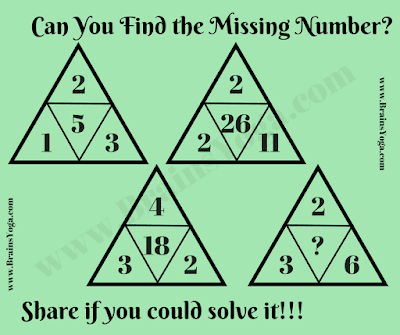## Wednesday, October 14, 2020

Here is the Maths Logic Picture Puzzle in which your challenge is to find the missing number. In this Picture Puzzle, 4 numbers are given in the Triangle which relates to each other with some pattern. To find this pattern and then find the value of missing number which will replace the question mark.Can you find the missing number?

The answer to this "Missing Number Maths Logic Puzzle", can be viewed by clicking on the button. Please do give your best try before looking at the answer.

1.1.Answer to this Maths Logic Missing Number puzzle is updated now.

2.I figured out the puzzle. The missing number is 12. I figured it out by deducing that the numbers in the center of the other groups are always mutiplied or added by the right number and the top. So I did 6X2 and got 12 of course.

2.1.3.4.5.6.